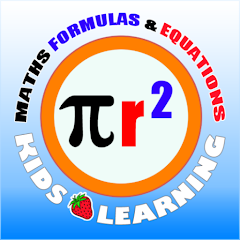# Maths Functions Flash Cards -

100+Everyone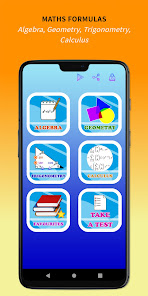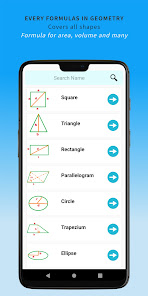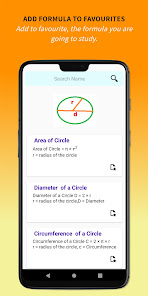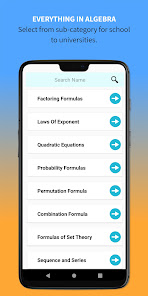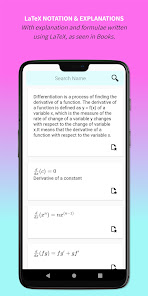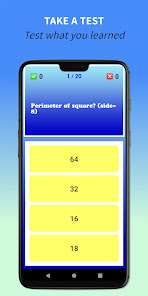Learn Maths Functions, Formulas & Equations with Flash Cards. Learn Geometry, Algebra, Trigonometry and Calculus formulae & Equations.

Maths Learning Flash Cards App provides all important formulas in mathematics. Arranged in very convenient sections and categories for all students from school to universities.

Sections
Geometry Mathematics Functions - Common formulas for finding area, perimeter, volume of all shapes including Square, Triangle, Rectangle, Parallelogram, Circle, Trapezium, Ellipse, Cube, Cylinder, Cone, Sphere, Hemisphere, Prism.
Algebra - Formulae for Factoring, Exponent, Quadratic Equation, Probability, Permutation, Sequence and Series, Complex Numbers, Binomial Theorem, Matrices, Combinations.
Trigonometry Mathematics Functions - Trigonometry Tables, Reciprocal, Inverse Trigonometry, Half Angle Identities, Double Angle, Triple Angle Identities, Periodicity.
Calculus Mathematical Formulas - Including Differential, Integral Calculus, Function Formulae, Limit Formulas, Derivative, Integral Formulas.

Add to Favourites and Search option
The app provides 100s of formulas and it will get difficult to learn from this huge set of formulas and equations. The app provies search option to quickly find what you a student is looking for.

Favourite List This allows each student to add the formulae and equations which is part of their current curriculum into a favourite list. This helps them to visit this section and revise only what they need for their studies.

LaTeX The app list all formulae using LaTeX notation which will be exactly how it appears on school text books and how they need to write it in exams.

Take a Test The app allows student to take a test to revise the formulae they have learned. This app is a learning app and not for testing a child's ability.
Updated on
Mar 7, 2023

## Data safety

Safety starts with understanding how developers collect and share your data. Data privacy and security practices may vary based on your use, region, and age. The developer provided this information and may update it over time.No data shared with third parties